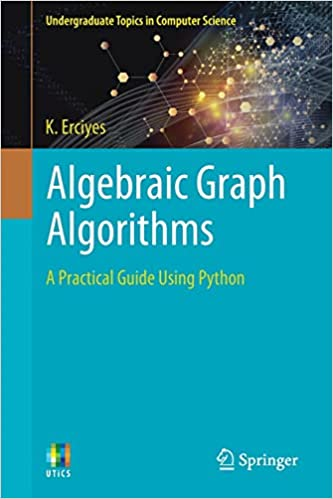Article info
• Views: 0
• Author: rukensai
• Date: 19-11-2021, 21:14
19-11-2021, 21:14

### Algebraic Graph Algorithms: A Practical Guide Using Python

Category: E-BooksEnglish | 2021 | ISBN: 3030878856 | 234 Pages | PDF EPUB | 27 MB

There has been unprecedented growth in the study of graphs, which are discrete structures that have many real-world applications. The design and analysis of algebraic algorithms to solve graph problems have many advantages, such as implementing results from matrix algebra and using the already available matrix code for sequential and parallel processing.
Providing Python programming language code for nearly all algorithms, this accessible textbook focuses on practical algebraic graph algorithms using results from matrix algebra rather than algebraic study of graphs. Given the vast theory behind the algebraic nature of graphs, the book strives for an accessible, middle-ground approach by reviewing main algebraic results that are useful in designing practical graph algorithms on the one hand, yet mostly using graph matrices to solve the graph problems. Python is selected for its simplicity, efficiency and rich library routines; and with the code herein, brevity is forsaken for clarity.

Topics and features:

​Represents graphs by algebraic structures, enabling new, robust methods for algorithm analysis and design
Provides matroid-based solutions to some graph problems, including greedy algorithm problems
Offers Python code that can be tested and modified for various inputs
Supplies practical hints, where possible, for parallel processing associated with algebraic algorithms
Links to a web page with supportive materials
This clearly arranged textbook will be highly suitable for upper-level undergraduate students of computer science, electrical and electronic engineering, bioinformatics, and any researcher or person with background in discrete mathematics, basic graph theory and algorithms.

Dr. Kayhan Erciyes is a full Professor in the Department of Software Engineering at Maltepe University, Istanbul, Turkey. His other publications include the Springer titles Discrete Mathematics and Graph Theory, Distributed Real-Time Systems, Guide to Graph Algorithms, Distributed and Sequential Algorithms for Bioinformatics, and Distributed Graph Algorithms for Computer Networks.